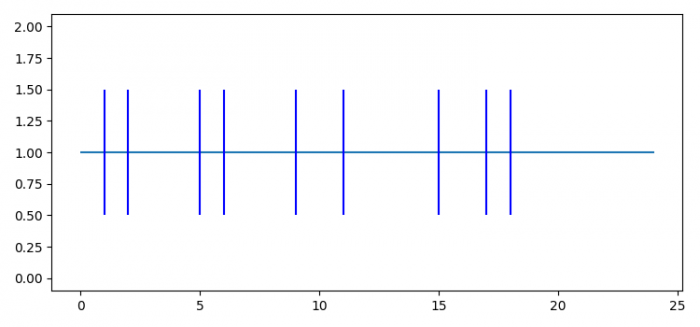# How to plot events on time using Matplotlib?

To plot events on time using Matplotlib, we can take the following steps −

• Set the figure size and adjust the padding between and around the subplots
• Make a list of data points, where event could occur.
• Plot a horizontal line with y, xmin and xmax.
• Plot identical parallel lines at the given positions.
• To display the figure, use show() method.

## Example

import matplotlib.pyplot as plt

plt.rcParams["figure.figsize"] = [7.50, 3.50]
plt.rcParams["figure.autolayout"] = True

a = [1, 2, 5, 6, 9, 11, 15, 17, 18]

plt.hlines(1, 0, 24)

plt.eventplot(a, orientation='horizontal', colors='b')

plt.show()

## Output